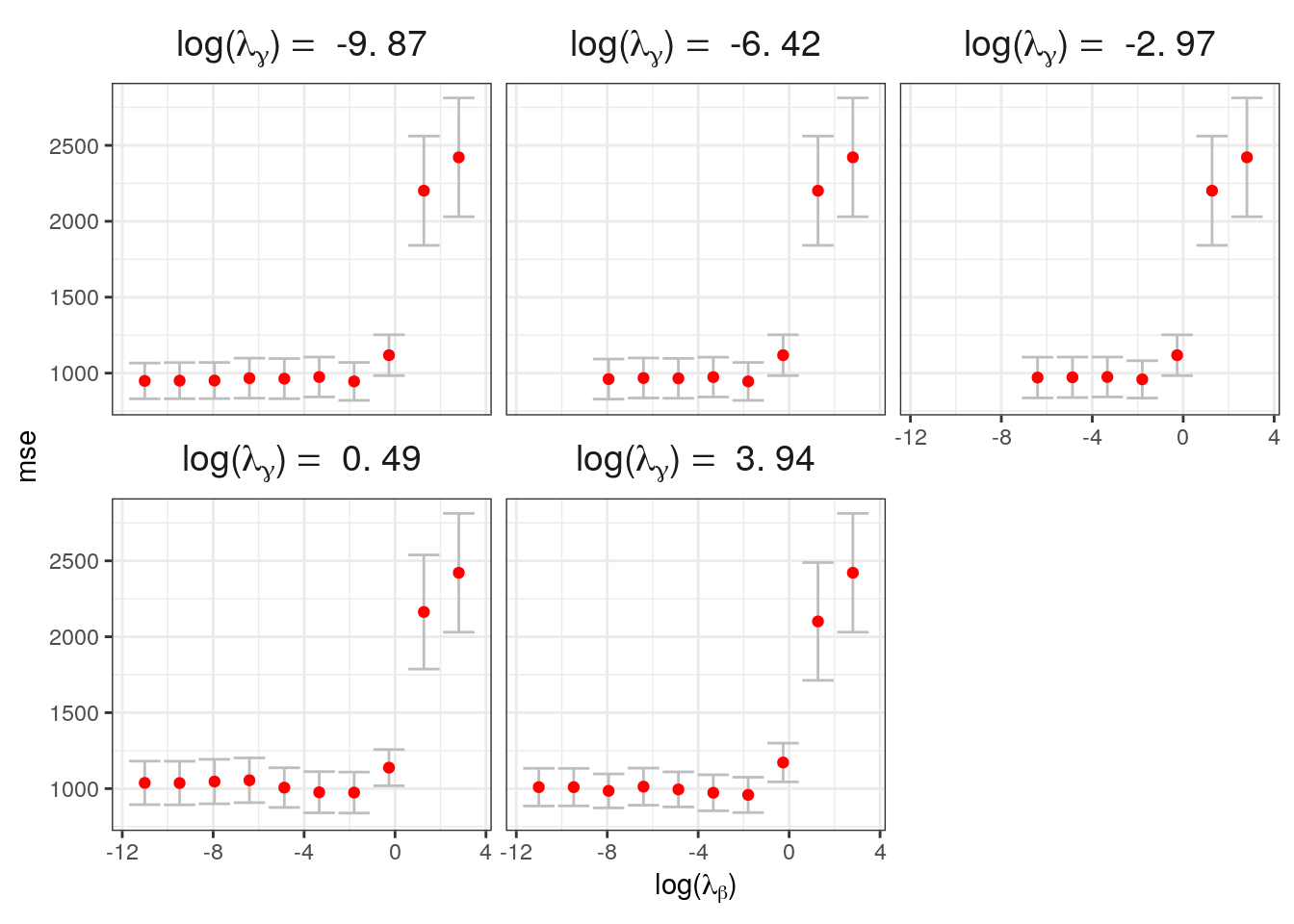# Math Expressions with Facets in ggplot2

In this post I show how we can use $\LaTeX$ math expressions to label the panels in facets.

The updated version of ggplot2 V 2.0 has improved the way we can label panels in facet plots with the use of a generic labeller function. The latex2exp package has made it much easier to write $\LaTeX$ expressions in R.

You will need to load the following packages for the code below to work:

I have posted some sample data in a GitHub Gist which you can import into your R session using the source_gist function from the devtools package:

pacman::p_load(devtools)
data <- devtools::source_gist(id = "ed3caf50247cae8e3e1c", filename = "facet_data.R")$value ## Sourcing https://gist.githubusercontent.com/sahirbhatnagar/ed3caf50247cae8e3e1c/raw/39df84c05eb9ac518c687c88f938dc4401468f60/facet_data.R ## SHA-1 hash of file is 3073e2ea147ce2818fa4e8841c6efa227378e1aa Then we create a labelling function which takes as input a string and prepends $\log(\lambda_{\gamma})$ to it. Note that latex2exp::TeX is the workhorse function that parses $\LaTeX$ syntax so that R understands it. Otherwise it becomes very messy to try and write more complex math expressions in R. appender <- function(string) TeX(paste("$\\log(\\lambda_{\\gamma}) = $", string))  The code to produce the plot is given by: ggplot(data, aes(log(lambda.beta), ymin = lower, ymax = upper)) + geom_errorbar(color = "grey") + geom_point(aes(x = log(lambda.beta), y = mse), colour = "red") + theme_bw() + facet_wrap(~lg, scales = "fixed", labeller = as_labeller(appender, default = label_parsed)) + theme(strip.background = element_blank(), strip.text.x = element_text(size = 14)) + xlab(TeX("$\\log(\\lambda_{\\beta})\$"))Note that we need to provide the default = label_parsed argument to the facet_wrap function so that it interprets the result from the appender function as math expressions.